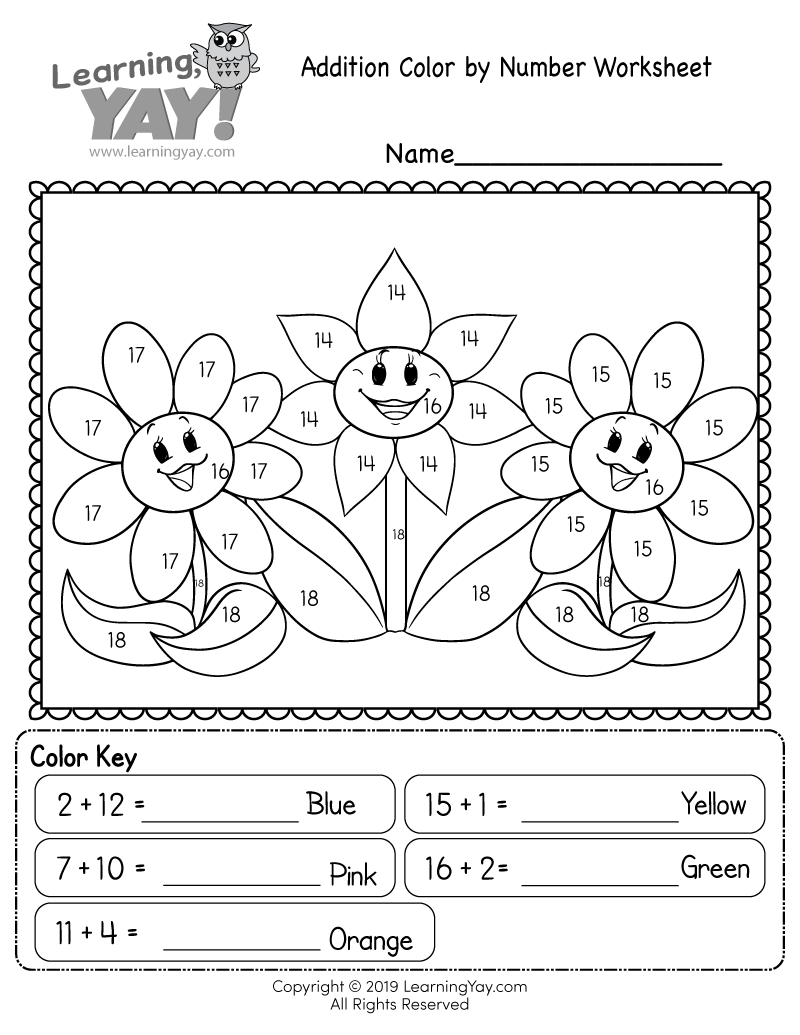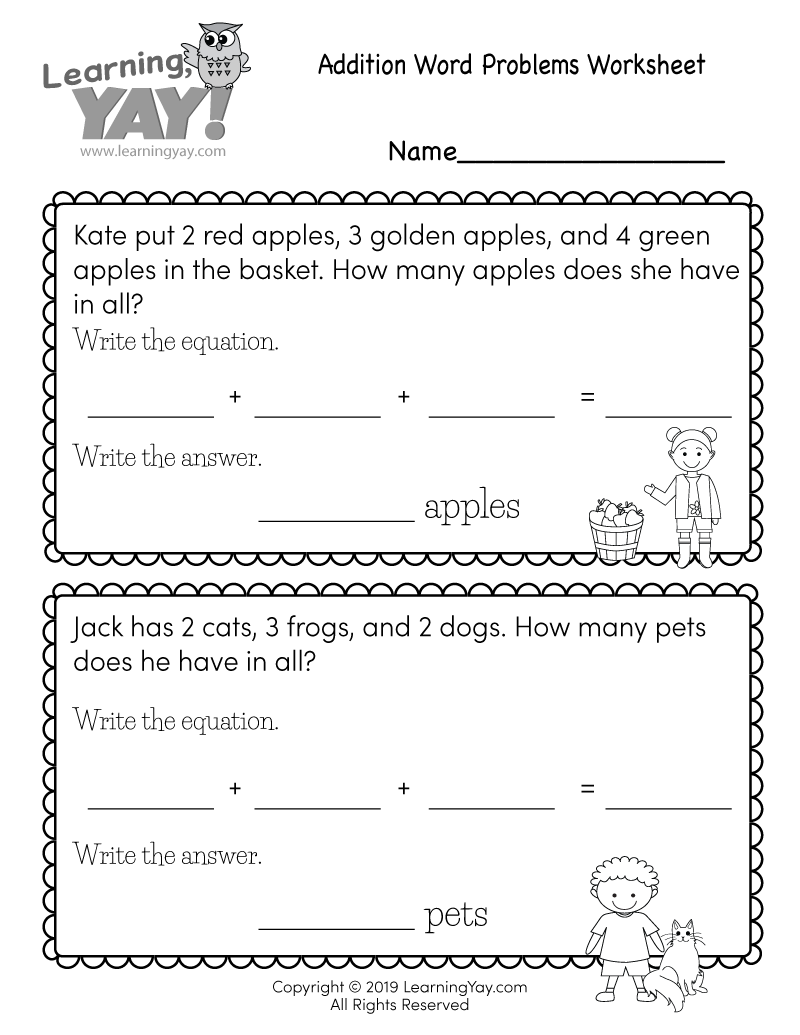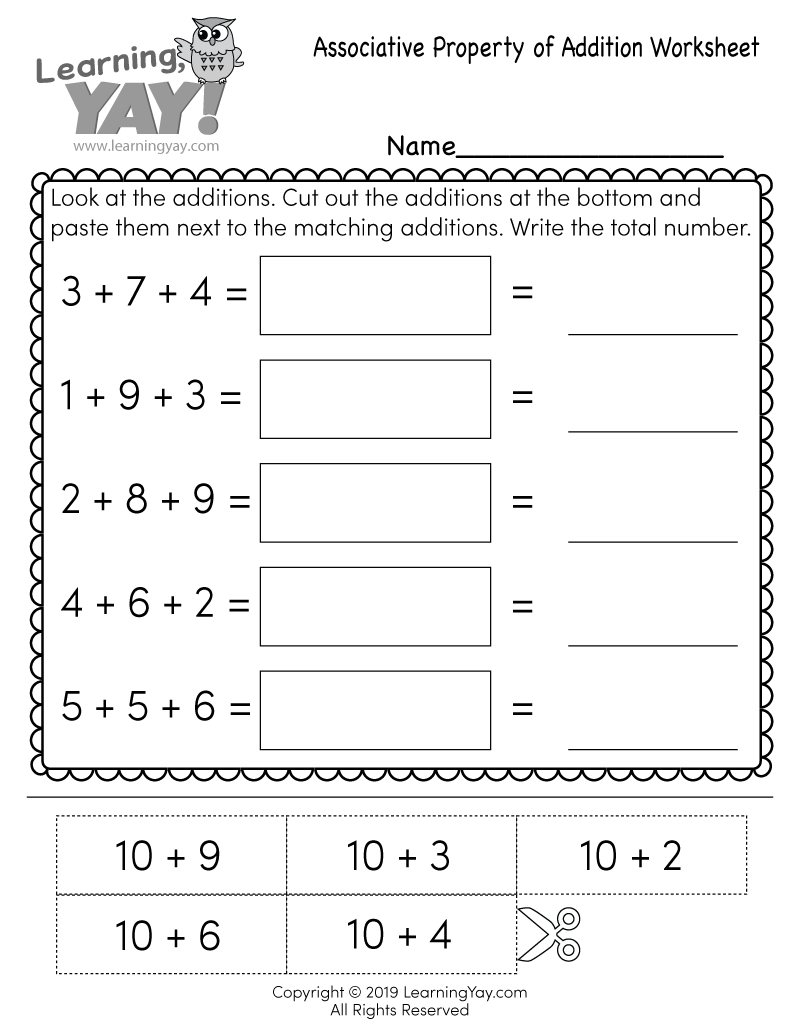# Addition Color by Number Worksheet

Students have to solve single and double-digit addition problems in order to complete a cute math coloring page that features three happy flowers. It is a fun way for first graders to practice adding numbers within 20.This free printable math worksheet is aligned to first grade Common Core standards. It is an excellent resource for teachers and parents who are teaching the following standard.
##### 1.OA.C.6 - Common Core ID

Add and subtract within 20, demonstrating fluency for addition and subtraction within 10. Use strategies such as counting on; making ten (e.g., 8 + 6 = 8 + 2 + 4 = 10 + 4 = 14); decomposing a number leading to a ten (e.g., 13 – 4 = 13 – 3 – 1 = 10 – 1 = 9); using the relationship between addition and subtraction (e.g., knowing that 8 + 4 = 12, one knows 12 – 8 = 4); and creating equivalent but easier or known sums (e.g., adding 6 + 7 by creating the known equivalent 6 + 6 + 1 = 12 + 1 = 13). Work with addition and subtraction equations.

Common Core » 1st Grade Math Standards » Operations & Algebraic Thinking » Add and subtract within 20. » 1.OA.C.6

## There are multiple ways to get this addition worksheet.

### You might also like these math worksheets: